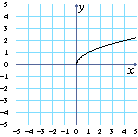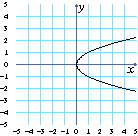# Sets, Relations, and Functions

A set is a collection of numbers or other mathematical objects, without repetitions. Any set of points in a table or on a graph is called a relation. Some relations are functions. In this lesson you will learn the difference between relations and functions. You will also learn about the domain and range of relations and functions.

## Sets

A set is a collection of numbers or other mathematical objects, each of which occurs only once. We write sets using curly braces. For example, \$\{2, 3, 4\}\$ is the set which contains the numbers 2, 3, and 4.

 What is the largest number in the set \$\{2, 3, 4\}\$?

You can list a set’s elements in any order. For example, \$\{1, 3, 5\}\$ and \$\{5, 1, 3\}\$ are the same set.

 What is the smallest number in the set \$\{5, 1, 3\}\$?

Sometimes, instead of listing everything contained in a set, we want to give some condition that the numbers in the set have to satisfy. For example, we might want to talk about the set of all positive numbers (numbers greater than zero). The notation for this is \$\{x: x > 0\}\$, which you should read as “the set of all \$x\$ such that \$x\$ is greater than 0” or “the set of all \$x\$ which are greater than 0.”

 Is the number 3 in the set \$\{x: x > 0\}\$?
 Is the number \$-2\$ in the set \$\{x: x ≤ -3\}\$?
 Is the number \$5\$ in the set \$\{x: -2 ≤ x ≤ 7\}\$? (\$-2 ≤ x ≤ 7\$ means \$-2 ≤ x\$ and \$x ≤ 7\$ are both true.)

## Relations

A relation is a set of ordered pairs \$(x,y)\$. If \$x\$ and \$y\$ are numbers, we can think of the ordered pair \$(x,y)\$ as the coordinates of a point in the plane, and a relation as a set of points in the plane.

We can also think of a relation as a two column “lookup table” of rows \$(x,y)\$, which takes inputs to outputs. Given an input \$x\$, you “look up” that value in the first column of the table, and any matching row gives an output \$y\$ in the second column. The set of inputs (\$x\$-values) of a relation is called the domain of the relation. The set of the outputs (\$y\$-values) of a relation is called the range of the relation.

The points on the grid to the left and the table to the right both represent the same relation. By looking at the table, you can see that the domain of the relation is the set \$\{-1,1,2,3,4\}\$. What is the range of this relation?
Range:
\$x\$\$y\$
\$-1\$\$1\$
\$1\$\$-1\$
\$2\$\$3\$
\$3\$\$-2\$
\$4\$\$2\$
Click to see a graph of the next relation. Find the domain and range of this relation using the table to the right. Remember that you don’t need to repeat numbers in a set.
 Domain: Range:
\$x\$\$y\$
\$-2\$\$0\$
\$-1\$\$1\$
\$-1\$\$-1\$
\$0\$\$2\$
\$0\$\$-2\$
\$1\$\$1\$
\$1\$\$-1\$
\$2\$\$0\$
Click and find the domain and range of this relation.
 Domain: Range:
\$x\$\$y\$
\$-2\$\$2\$
\$-1\$\$2\$
\$0\$\$2\$
\$1\$\$2\$
\$2\$\$2\$

Sometimes instead of a table you have to look at a graph to find the domain and range of a relation. Look at the grid to the left. This time the graph is not just a few points, but the collection of points that make the red curve.

Click or tap inside the grid and drag the vertical bar. You see that the curve goes from \$x=-2\$ to \$x=4\$. So the domain of this relation is \$\{x:-2≤x≤4\}\$. Within that domain, the curve goes from \$y=-1\$ to \$y=2\$. What is the range of this relation?

Range:

Click and find the domain and range of this new relation using its graph.

 Domain: Range:

Look at the graph of \$y=x^2\$. It seems that the curve goes from \$x=-3\$ to \$x=3\$. Click or tap inside the grid and drag the vertical bar to \$x=4\$. To the right of the grid you see that \$y=16\$, so even though we can’t see it on this grid, there is a point on the graph with \$x\$-coordinate 4.

 Drag the vertical bar to \$x=-5\$. Is there a point on the graph with \$x\$-coordinate \$-5\$?
 If you zoomed out far enough, would there be a point on the graph with \$x\$-coordinate 40?
 Click to look at more of the graph. Does this match your previous answer?
 Is there a point on the graph with \$x=-25000\$?
 Is there a limit to how large or small the \$x\$-value of a point can be on the graph of \$y=x^2\$?
 When there is no limit on what \$x\$ can be, we say that the domain of a relation is “all real numbers”. What is the domain of \$y=x^2\$?
 What is the smallest \$y\$-coordinate of any point on this curve?

What is the range of this relation?

Range:

## Functions

A relation is called a function if there is exactly one output (\$y\$-value) for each input (\$x\$-value). If a relation has two or more outputs for a single input, it is not a function. For the two relations shown below, the one on the right isn’t a function because there are two outputs for each input greater than 0. The relation on the left is a function.A Function Not a Function

A relation is graphed on the grid to the left. The trace (vertical bar) is currently at \$x=3\$, allowing you to see that the point \$(3,0)\$ is on the graph.

 Click or tap inside the grid to the left and drag the vertical bar to place it on the marked points on the graph. What are the coordinates of these points?
 What is the input (\$x\$-value) for each of the two points in the previous question?
 How many outputs (\$y\$-values) are there for the input (\$x\$-value) you found in the previous question?
 Is the relation shown to the left a function?

What is the domain of this relation?

Domain:

 Click . Use the vertical bar in the grid to decide if the relation is a function. Is it a function?

The domain of this relation is all real numbers. What is its range?

Range:

Click . Use the vertical bar in the grid to decide if the relation is a function and to help find its domain and range.

 Function? Domain: Range:
 Click . Is this relation a function?
You can also decide if a relation is a function by looking at a table. In the table to the right the input \$-2\$ has two outputs (1 and \$-1\$). You can also see this by clicking on the graph of the relation: there are two points on that graph that have \$x\$-coordinate \$-2\$.
 Find another input in the table that has more than one output.
 Is this relation a function?
\$x\$\$y\$
\$-4\$\$3\$
\$-3\$\$2\$
\$-2\$\$1\$
\$-2\$\$-1\$
\$-1\$\$0\$
\$0\$\$-1\$
\$1\$\$-2\$
\$1\$\$3\$
\$2\$\$-3\$
 Are there any inputs in the table that have two outputs (or are there two points with the same \$x\$-coordinate)?
 Is this relation a function?
\$x\$\$y\$
\$-5\$\$4\$
\$-4\$\$3\$
\$-3\$\$2\$
\$-2\$\$1\$
\$-1\$\$0\$
\$0\$\$-1\$
\$1\$\$0\$
\$2\$\$1\$
\$3\$\$2\$
\$4\$\$3\$
\$5\$\$4\$
 Click and use the table or the graph to decide if this relation is a function. Is it a function?
\$x\$\$y\$
\$-5\$\$1\$
\$-4\$\$-1\$
\$-3\$\$1\$
\$-2\$\$-1\$
\$-1\$\$1\$
\$0\$\$-1\$
\$1\$\$1\$
\$2\$\$-1\$
\$3\$\$1\$
\$4\$\$-1\$
\$5\$\$1\$